#### Explained with examplesPhoto by Tetiana SHYSHKINA on Unsplash

What are the average house prices in different cities of the US? What are the total sales amounts of different product groups in a store? What are the average salaries in different companies?

All these questions can be answered by using a grouping operation given that we have proper data. Most data analysis libraries and frameworks implement a function to perform such operations.

In this article, we will compare two of the most popular data analysis libraries with regards to tasks that involve grouping. The first one is Python Pandas and the other is R data table.

We will be using the Melbourne housing dataset available on Kaggle for the examples. We first import the libraries and read the dataset.

`# pandasimport pandas as pdmelb = pd.read_csv("/content/melb_data.csv")`
`# data.tablelibrary(data.table)melb <- fread("datasets/melb_data.csv")`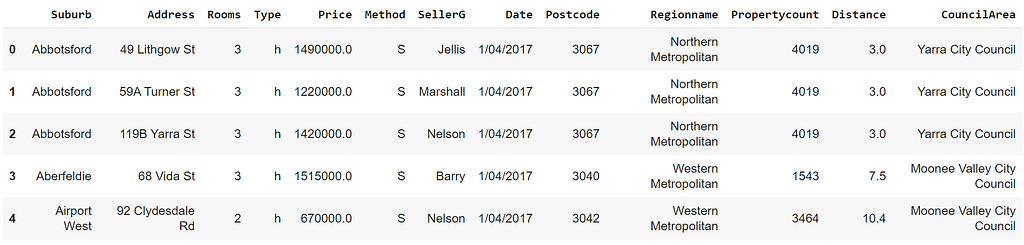The first 5 rows the data (image by author)

Let’s start with finding the average number of rooms for each house type. Pandas provides the groupby function for grouping the observations (i.e. rows).

`# pandasmelb[["Type","Rooms"]].groupby("Type").mean()`
`        RoomsTypeh    3.405189t    2.944260u    2.061948`

We select both the type and room columns and group the houses (i.e. rows) by the type column. Since there are 3 distinct values in the type column, we end up having 3 groups. Finally, we apply the mean function to each group and get the average number of rooms.

If we do not select the columns and directly apply the groupby function, Pandas calculates the average values for all numerical columns. There is a more practical way to overcome this issue which we will cover in the following examples.

Here is how we do the same operation with data table:

`# data.tablemelb[, .(mean(Rooms)), by=Type]`
`   Type       V11:    h 3.4051892:    t 2.9442603:    u 2.061948`

The intuition is the same but the syntax is different. The by parameter is used for selecting the column to be used for grouping. Data table has a way of separating different types of operations with commas. For instance, if we need to pass a condition to filter rows, we place it before the first comma inside the square brackets.

For the second example, we calculate the average house prices in each region and assign a name to the aggregated column.

`# pandasmelb.groupby("Regionname", as_index=False).agg(    avg_house_price = ("Price", "mean"))`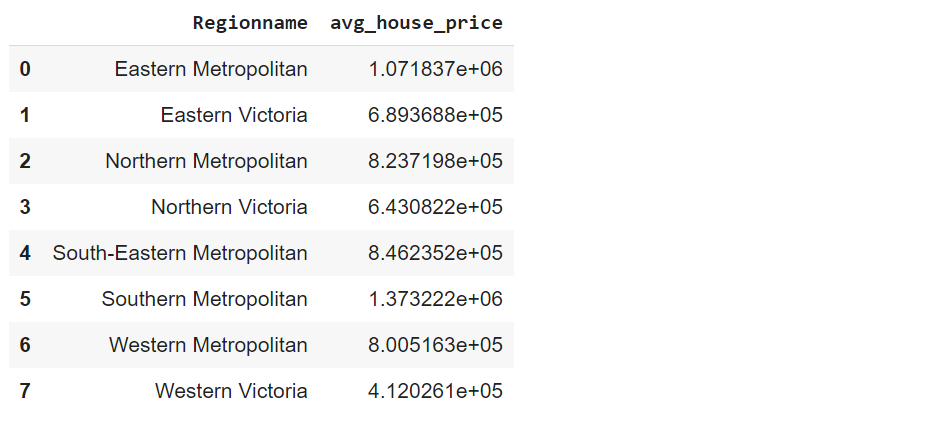(image by author)

We have used a named aggregation this time so we did not have to select any columns. The type of aggregation and the column to be aggregated are specified inside the agg function. We can also assign a customized name to the aggregated column.

The as_index parameter is used for creating a column for the groups. Otherwise, they are represented as the index of the data frame.

Here is the data table version:

`# data.tablemelb[, .(avg_house_price = mean(Price, na.rm = TRUE)), by=Regionname]`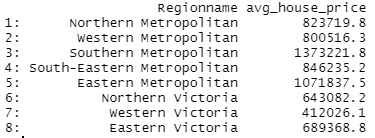(image by author)

The data table syntax does not change much. We have just added the na.rm parameter because there are missing values in the price column. We need to remove them before calculating the average. Otherwise, all aggregated values become NA.

Both libraries allow for nested groupings so we can group the observations based on multiple columns. Let’s find the average house prices for each type in each region.

`# pandasmelb.groupby(["Regionname", "Type"], as_index=False).agg(   avg_house_price = ("Price", "mean"))[:5]`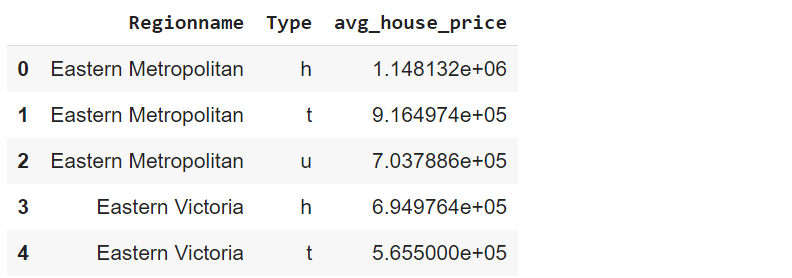(image by author)

We use a list to pass multiple columns to the groupby function. The 5 at the end of the code limits the number of rows to be displayed.

`# data.tablemelb[, .(avg_house_price = mean(Price, na.rm = T)), .(Regionname, Type)][1:5]`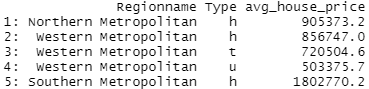(image by author)

As you may have noticed, we do not have to use the by keyword. The standard structural syntax of data table allows it to know which columns are used for grouping.

Just like we can group by multiple columns, we can calculate multiple aggregations for each group. Furthermore, they do not have to be the same type of aggregation.

For instance, we can calculate the average number of rooms for each house type and count the number of houses in each group.

`# pandasmelb.groupby("Type", as_index=False).agg(   avg_number_of_rooms = ("Rooms", "mean"),   number_of_houses = ("Rooms", "count"))`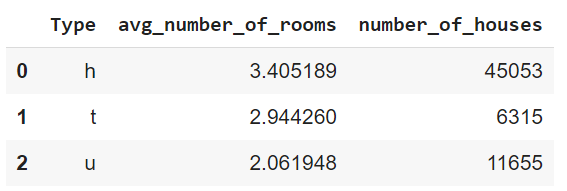(image by author)
`# data.tablemelb[,     .(avg_number_of_rooms = mean(Rooms), number_of_houses = .N)     , Type]`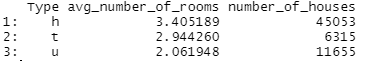(image by author)

Let’s finish up with a slightly more complicated example. We first filter the observations (i.e. rows) based on a condition and then apply the grouping operation. Finally, we sort the results based on an aggregated column.

`# pandasmelb[melb.Price > 1000000].groupby("Type").agg(   avg_distance = ("Distance", "mean"),   number_of_houses = ("Distance", "count")).sort_values(by="avg_distance")`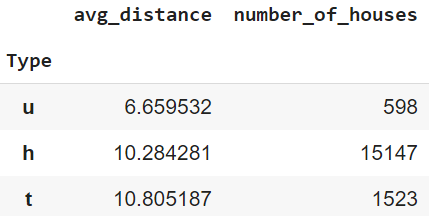(image by author)
`# data.tablemelb[Price > 1000000,     .(avg_distance = mean(Distance),       number_of_houses = .N)     , Type][order(avg_distance)]`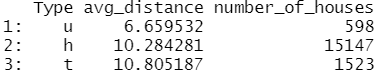(image by author)

The filtering is the first operation as expected. The sort_values and order functions do the sorting for pandas and data table, respectively. They both sort in ascending order by default. To sort in descending order, we can set the ascending parameter as false for pandas. Data table sorts in descending order if we add a minus sign before the column name.

#### Conclusion

Grouping observations based on distinct values or categories in a column and then apply some aggregations is of crucial importance for exploratory data analysis.

Thus, data analysis and manipulation libraries provide flexible functions to handle such operations. We have done several examples that demonstrate how grouping is done with pandas and data table libraries.

Thank you for reading. Please let me know if you have any feedback.How Grouping Works with Python Pandas vs R Data Table was originally published in Towards Data Science on Medium, where people are continuing the conversation by highlighting and responding to this story.